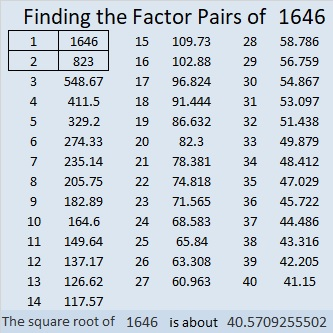# 1646 Mystery Level

Contents

### Today’s Puzzle:

It’s a mystery if this puzzle is easy, difficult, or half easy and half difficult. The only way for you to know is to use logic to try solving it yourself!### Factors of 1646:

• 1646 is a composite number.
• Prime factorization: 1646 = 2 × 823.
• 1646 has no exponents greater than 1 in its prime factorization, so √1646 cannot be simplified.
• The exponents in the prime factorization are 1 and 1. Adding one to each exponent and multiplying we get (1 + 1)(1 + 1) = 2 × 2 = 4. Therefore 1646 has exactly 4 factors.
• The factors of 1646 are outlined with their factor pair partners in the graphic below.### More About the Number 1646:

823 is part of the prime decade: 821, 823, 827, 829.
You can be sure that each of those primes doubled: 1642, 1646, 1654, and 1658 will have exactly four factors.

1646 is in only one Pythagorean triple:
1646-677328-677330, calculated from 2(823)(1), 823² – 1², 823² + 1².

This site uses Akismet to reduce spam. Learn how your comment data is processed.